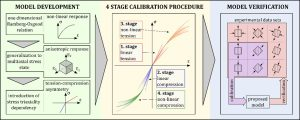Researchers at the Laboratory for Numerical Modelling and Simulation (LNMS) developed a new constitutive model for describing fibre-reinforced composite materials. The model covers non-linear, anisotropic and tension-compression asymmetric material behaviour. The model is published in the International Journal of Engineering Science (IF=7.155).

The model is based on the Ramberg-Osgood relation, which is generalized to multiaxial anisotropic form via strain energy potential. Stress triaxiality dependency is then introduced to implement asymmetric material response. The model covers all fundamental properties for describing the elastic behaviour of fibre-reinforced composites. Novelty in this model is the method of implementing asymmetric response, which offers a high degree of flexibility and opens numerous possibilities for developing similar models.

Material model response is defined by four fourth-order tensors, which can be determined separately with four stage calibration procedure. An advantage of this model is a simple calibration procedure, where only classic uniaxial tension and compression tests suffice. The model is implemented in ABAQUS/Explicit finite element method software. For the verification, two experimental data sets are used, where the model, calibrated from uniaxial tests, excellently predicted material response in other stress states.Figure 1: Model development, calibration and verification procedure.